# 漫画：Integer 竟然有 6 种比较方式？﻿﻿﻿﻿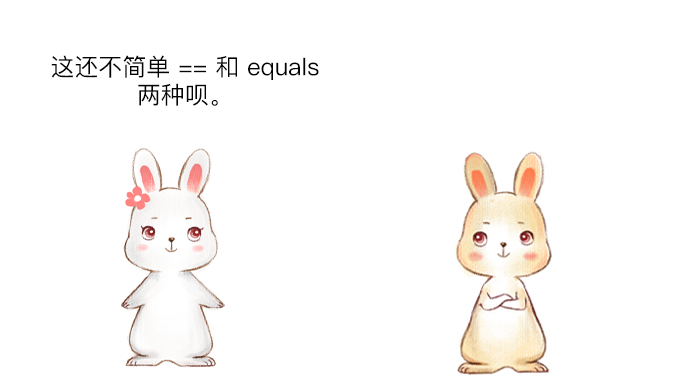﻿﻿﻿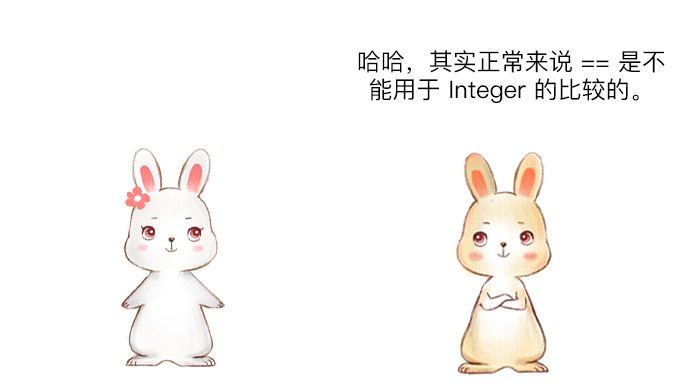﻿﻿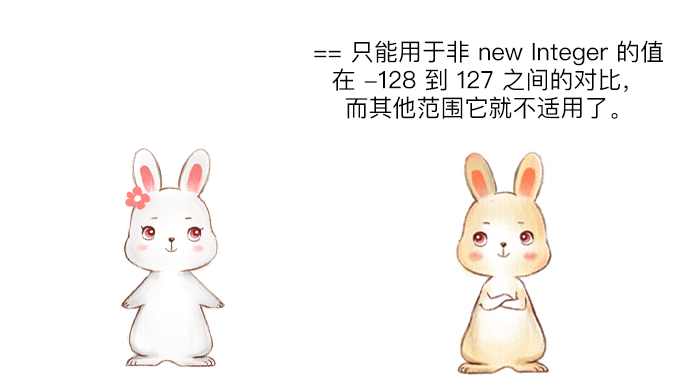﻿﻿﻿

## 代码测试

public class IntegerTest {    public static void main(String[] args) {        Integer i1 = 127;        Integer i2 = 127;        System.out.println(i1 == i2);        Integer i3 = 128;        Integer i4 = 128;        System.out.println(i3 == i4);    }}

true

false

﻿﻿﻿﻿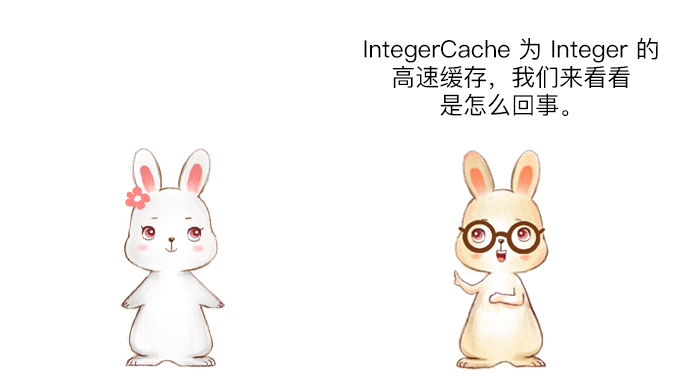﻿

public class IntegerTest {  public static void main(String[] paramArrayOfString) {    Integer integer1 = Integer.valueOf(127);    Integer integer2 = Integer.valueOf(127);    System.out.println((integer1 == integer2));    Integer integer3 = Integer.valueOf(128);    Integer integer4 = Integer.valueOf(128);    System.out.println((integer3 == integer4));  }}

public static Integer valueOf(int i) {    if (i >= IntegerCache.low && i <= IntegerCache.high)        return IntegerCache.cache[i + (-IntegerCache.low)];    return new Integer(i);}

private static class IntegerCache {    static final int low = -128;    static final int high;    static final Integer cache[];    static {        // high value may be configured by property        int h = 127;        String integerCacheHighPropValue =            sun.misc.VM.getSavedProperty("java.lang.Integer.IntegerCache.high");        if (integerCacheHighPropValue != null) {            try {                int i = parseInt(integerCacheHighPropValue);                i = Math.max(i, 127);                // Maximum array size is Integer.MAX_VALUE                h = Math.min(i, Integer.MAX_VALUE - (-low) -1);            } catch( NumberFormatException nfe) {                // If the property cannot be parsed into an int, ignore it.            }        }        high = h;        cache = new Integer[(high - low) + 1];        int j = low;        for(int k = 0; k < cache.length; k++)            cache[k] = new Integer(j++);        // range [-128, 127] must be interned (JLS7 5.1.7)        assert IntegerCache.high >= 127;    }    private IntegerCache() {}}

﻿

【强制】所有整型包装类对象之间值的比较，全部使用 equals 方法比较。

#### 注意事项

public class IntegerTest {    public static void main(String[] args) {        Integer i1 = new Integer(127);        Integer i2 = new Integer(127);        System.out.println(i1 == i2);    }}

false

﻿

﻿

﻿﻿﻿

## 其他比较方式

### compareTo

public final class Integer extends Number implements Comparable<Integer> { // 忽略其他内容}

compareTo 的使用如下：

public class IntegerTest {    public static void main(String[] args) {        Integer i1 = new Integer(128);        Integer i2 = new Integer(128);        System.out.println(i1.compareTo(i2));    }}

0

compareTo 的源码如下：

public int compareTo(Integer anotherInteger) {    return compare(this.value, anotherInteger.value);}public static int compare(int x, int y) {    return (x < y) ? -1 : ((x == y) ? 0 : 1);}

### 直接运算

compareTo 方法给我们了一个启发，我们可以直接将两个值进行相减，如果相减的值等于 0，则说明对比的两个值是相同的，示例代码如下：

public class IntegerTest {    public static void main(String[] args) {        Integer i1 = new Integer(128);        Integer i2 = new Integer(128);        System.out.println((i1 - i2) == 0);    }}

true

### intValue

public class IntegerTest {    public static void main(String[] args) {        Integer i = 558;        Integer i2 = 558;        System.out.println(i.intValue() == i2.intValue());    }}

true

### 异或

• 1 异或 0=1

• 0 异或 0=0

• 1 异或 1=0

public class IntegerTest {    public static void main(String[] args) {        Integer i = 558;        Integer i2 = 558;        System.out.println((i ^ i2) == 0);    }}

true

## 扩展知识：IntegerCache 值域修改

IntegerCache 默认的取值范围为 -128 到 127，但我们可以通过设置启动参数来调整 IntegerCache 的最大缓存值，比如我们可以配置虚拟机的启动参数 -XX:AutoBoxCacheMax=1000，此配置表示将缓存的最大值设置为 1000，如果是 Idea 的配置如下：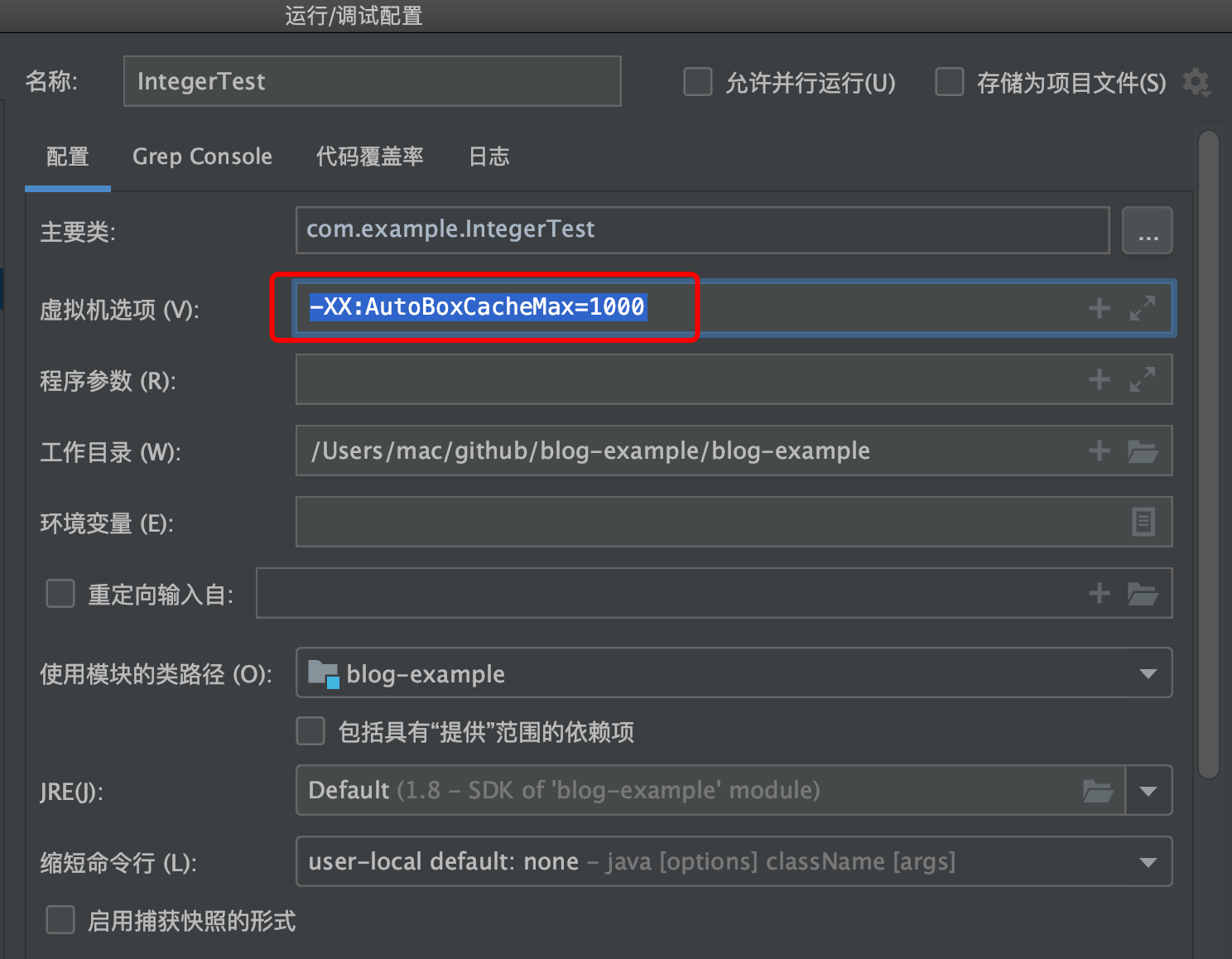public class IntegerTest {    public static void main(String[] args) {        Integer i1 = 999;        Integer i2 = 999;        System.out.println(i1 == i2);    }}

true

﻿

## 总结

﻿﻿﻿﻿

## 互动话题

﻿## 评论 (1 条评论)2020 年 07 月 08 日 10:00